# Introduction

Here is a quick data-scientist / data-analyst question: what is the overall trend or shape in the following noisy data? For our specific example: How do we relate `value` as a noisy function (or relation) of `m`? This example arose in producing our tutorial “The Nature of Overfitting”.

One would think this would be safe and easy to asses in `R` using `ggplot2::geom_smooth()`, but now we are not so sure.

# Our Example

Let’s first load our data and characterize it a bit

``````d <- read.csv(
'sus_shape.csv',
strip.white = TRUE,
stringsAsFactors = FALSE)

``````##   m      value
## 1 3 -12.968296
## 2 3  -5.522812
## 3 3  -6.893872
## 4 3  -5.522812
## 5 3 -11.338718
## 6 3 -10.208145``````
``summary(d)``
``````##        m              value
##  Min.   :   3.0   Min.   :-18.773
##  1st Qu.:  86.0   1st Qu.: -1.304
##  Median : 195.0   Median : -1.276
##  Mean   : 288.8   Mean   : -1.508
##  3rd Qu.: 436.0   3rd Qu.: -1.266
##  Max.   :1000.0   Max.   : -1.260``````
``nrow(d)``
``##  15545``

Now let’s try and look at this data. First we try a scatter plot with a low alpha, which gives us something similar to a density presentation.

``````library(ggplot2)

ggplot(
data = d,
mapping = aes(x = m, y = value)) +
geom_point(
alpha = 0.005,
color = 'Blue') +
ggtitle("point plot of data")``````Each `m` value has many different `value` measurements (representing repetitions of a noisy experiment). Frankly the above is not that legible, so we need tools to try and summarize it in the region we are interested in (`value` near `-1.25`).

# Trying Default Smoothing

Let’s run a default smoothing line through this data to try to get the overall relation.

``````ggplot(
data = d,
mapping = aes(x = m, y = value)) +
geom_smooth() +
ggtitle("suspect shape in smoothing (default)")``````
``## `geom_smooth()` using method = 'gam' and formula 'y ~ s(x, bs = "cs")'``This graph appears to imply some sort of oscillation or structure in the relation between mean `value` and `m`. We are pretty sure there is no such structure, and this is an artifact of the smoothing method. This defect is why we did not use `ggplot2::geom_smooth()` in our note on training set size.

We did see a warning, but we believe this is just telling us which default values were used, and not indicating the above pathology was detected.

At this point we are in a pickle. We had theoretical reasons to believe the data is a monotone increasing in `m` trend, with mean-zero noise that decreases with larger `m`. The graph doesn’t look like that. So our understanding or theory could be wrong, or the graph didn’t faithfully represent the data. The graph had been intended as a very small step in larger work. Re-examining the intricacies of what is the default behavior of this graphing software was not our intended task. We had been doing some actual research on the data.

Now have a second problem: is this unexpected structure in our data, or a graphing artifact? The point is: when something appears to work one can, with some risk, move on quickly; when something appears to not work in a surprising way, you end up with a lot of additional required investigation. This investigation is the content of this note, like it or not. Also in some loud `R` circles, one has no choice but to try “the default `ggplot2::geom_smooth()` graph”, otherwise one is pilloried for “not knowing it.”

We can try switching the smoothing method to see what another smoothing method says. Let’s try `loess`.

``````ggplot(
data = d,
mapping = aes(x = m, y = value)) +
geom_smooth(method = 'loess') +
ggtitle("suspect shape in smoothing (loess)")``````
``## `geom_smooth()` using formula 'y ~ x'``Now we have a different shape. At most one of these (and in fact neither) is representative of the data. There is, again, a warning. It appears, again, to be a coding style guide- and not detection of the issue at hand.

# Looking Again

Let’s try a simple grouped box plot. We will group `m` into ranges to get more aggregation.

``````d\$m_grouped <- formatC(
round(d\$m/50)*50,
width = 4,
format = "d",
flag = "0")

ggplot(
data = d,
mapping = aes(x = m_grouped, y = value)) +
geom_boxplot() +
theme(axis.text.x = element_text(angle = 90,
vjust = 0.5,
hjust=1)) +
ggtitle("m-grouped bar chart, no obvious plotting artifacts.")``````For legibility, we repeat these graphs zooming in to the area under disagreement. We are using `coord_cartesian()` to zoom in, so as to try and not change the underlying graphing calculation.

``zoom <- coord_cartesian(xlim = c(0, 500), ylim = c(-1.5, -1)) ``
``````ggplot(
data = d,
mapping = aes(x = m, y = value)) +
geom_smooth() +
zoom +
ggtitle("suspect shape in smoothing (default, zoomed)") +
geom_hline(
yintercept = max(d\$value),
color = 'red',
linetype = 2)``````
``## `geom_smooth()` using method = 'gam' and formula 'y ~ s(x, bs = "cs")'``This crossing above `-1.0` is very suspicious, as we have `max(d\$value)` = -1.2600449. We have annotated this with the horizontal red dashed line.

And the entirety of the `loess` hump is also a plotting artifact, also completely out of the observed data range.

``````ggplot(
data = d,
mapping = aes(x = m, y = value)) +
geom_smooth(method = 'loess') +
zoom +
ggtitle("suspect shape in smoothing (loess, zoomed)") +
geom_hline(
yintercept = max(d\$value),
color = 'red',
linetype = 2)``````
``## `geom_smooth()` using formula 'y ~ x'``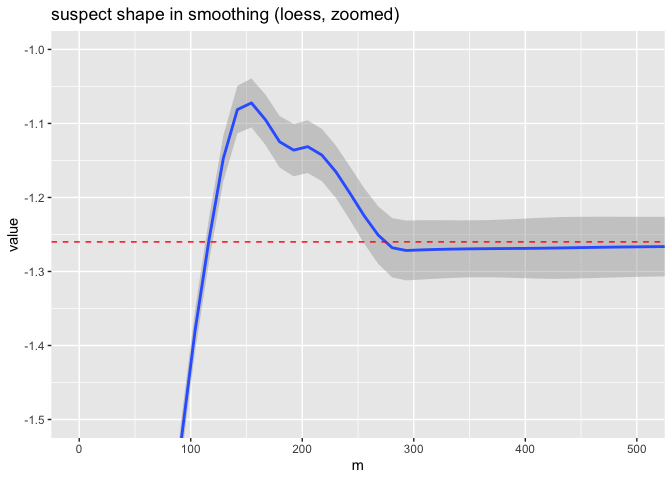The zoomed-in version of the box plot shows the noisy monotone asymptotic shape we expected for the original experiment that produced this data.

``````ggplot(
data = d[d\$m <= 500, ],
mapping = aes(x = m_grouped, y = value)) +
geom_boxplot() +
coord_cartesian(ylim = c(-1.5, -1.2)) +
theme(
axis.text.x = element_text(
angle = 90,
vjust = 0.5,
hjust=1)) +
ggtitle("m-grouped bar chart, no obvious plotting artifacts, zoomed")``````The point plot, when zoomed, qualitatively agrees with the boxplot.

``````ggplot(
data = d,
mapping = aes(x = m, y = value)) +
geom_point(alpha = 0.05, color = 'Blue') +
coord_cartesian(
xlim = c(0, 500),
ylim = c(-1.5, -1.25))  +
ggtitle("point plot of data, zoomed")``````# Directly calling `loess`/`lowess`

`ggplot2` is documented as using `loess`, which in turn is documented as a newer adapter for `lowess` “with different defaults” then `loess`. However, the documented exposed controls on these two methods seem fairly disjoint.

That being said `loess` (without a ‘w’, as in “Uruguay”) called directly with default arguments shows the same chimeric artifact.``zoom2 <- coord_cartesian(ylim = c(-1.5, -1)) ``
``````d\$loess <- loess(value ~ m, data = d)\$fitted

ggplot(
data = d,
mapping = aes(x = m)) +
geom_line(aes(y = loess)) +
geom_point(
aes(y = value),
alpha = 0.01,
color = 'Blue') +
zoom2 +
geom_hline(
yintercept = max(d\$value),
color = 'red',
linetype = 2) +
ggtitle('direct loess (no w) call')``````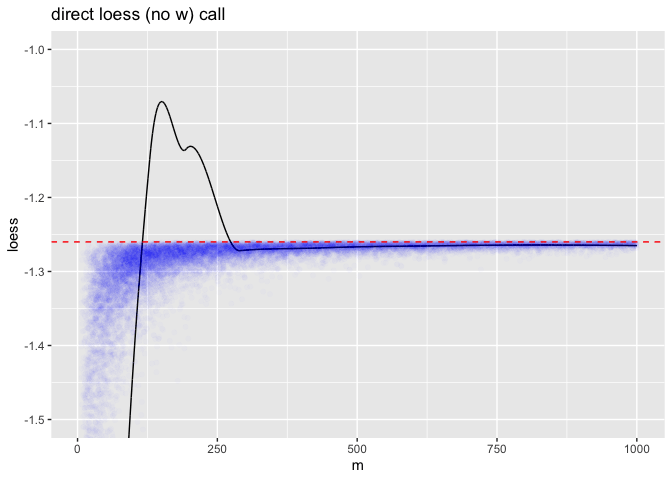Playing with arguments can suppress the artifact, but we still saw weird (but smaller) effects even with the suggested `degree = 1` alternate setting.

Directly calling `lowess` (with a ‘w’, as in “answer”) gives a more reasonable result out of the box.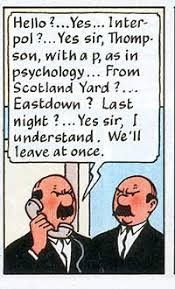``````d\$lowess <- lowess(d\$m, d\$value)\$y

ggplot(
data = d,
mapping = aes(x = m)) +
geom_line(aes(y = lowess)) +
geom_point(
aes(y = value),
alpha = 0.01,
color = 'Blue') +
geom_hline(
yintercept = max(d\$value),
color = 'red',
linetype = 2) +
coord_cartesian(
ylim = c(-1.5, -1.25)) +
ggtitle('direct lowess (with w) call')``````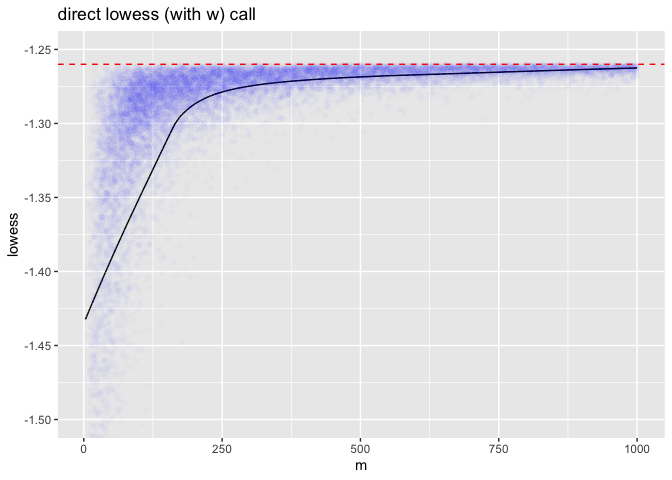# Simple Windowing

Simple methods from fields such as signal processing work well. For example, a simple square-window moving average appears to correctly tell the story. These are the methods I use, at the risk of being told I should have used `geom_smooth()`.

``````# requires development version 1.3.2
# remotes::install_github('WinVector/WVPlots')
library(WVPlots)  ``````
``## Loading required package: wrapr``
``````ConditionalSmoothedScatterPlot(
d,
xvar = 'm',
yvar = 'value',
point_color = "Blue",
point_alpha = 0.01,
k = 51,
groupvar = NULL,
title = 'Width 51 square window on data (zoomed)') +
coord_cartesian(ylim = c(-1.5, -1.25)) +
geom_hline(
yintercept = max(d\$value),
color = 'red',
linetype = 2)``````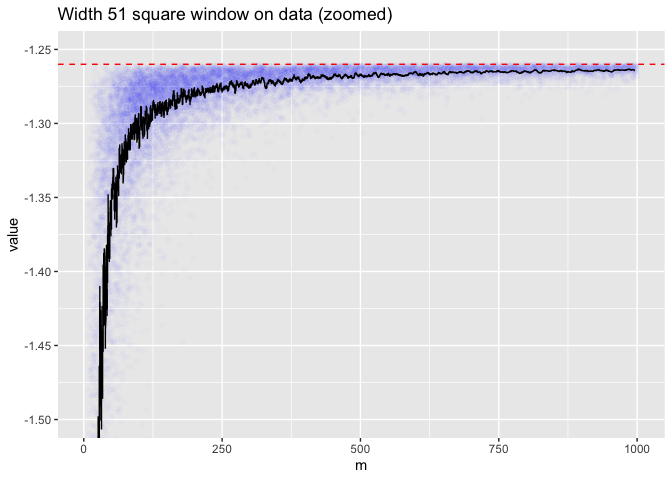The fact that the hard window yields a jagged curve gives an indication of the amount of noise in each region of the graph.

# Conclusion

Large data sets are inherently illegible. So we rely on summaries and aggregations to examine them. When these fail we may not always be in a position to notice the distortion, and this can lead to problems.

Many of the above default summary presentations were deeply flawed and showed chimerical artifacts not in the data being summarized. Starting a research project to understand the nature of the above humps and oscillations would be fruitless, as they are not in the data, but instead artifacts of the plotting and analysis software.

As a consultant this is disturbing: I end up spending time on debugging the tools, and not on the client’s task.

The above were not flaws in `ggplot2` itself, but in the use of the `gam` and `loess` smoothers, which are likely introducing the artifacts by trying to enforce certain curvature conditions not in the data. We are essentially looking at something akin to Gibbs’ phenomenon or ringing. This could trip up the data scientist or the data analyst without a background in signal analysis.

This sort of problem reveals the lie in the typical “data scientist >> statistician >> data analyst” or “statistics are always correct in `R`, and never correct in `Python`” snobberies. In fact a data analyst would get the summary shapes right, as presentation of this sort is one of their specialties.

Categories: Pragmatic Data Science Tutorials### jmount

Data Scientist and trainer at Win Vector LLC. One of the authors of Practical Data Science with R.

### 10 replies ›

1.Antony Unwin says:

Your zoomed point plot provides important information about the data. Perhaps if you had looked at that first, you would not have used these methods and had these problems. Graphical Data Analysis should come first (well, I would say that :-)).

Are the data real and available?

1.jmount says:

I did look at more than one graph, under the idea that one look is not always enough. The `WVPlots::ConditionalSmoothedScatterPlot` shown at the end is my go to for this sort of situation, it is a good compromise of legibility and faithfulness. In fact it is the summary graph family I use in the original article. This follow-up not is the set of visualizations I explored to confirm what visualization was legible and respected what was in the data.

The data is real and can be found here: https://github.com/WinVector/Examples/tree/main/The_Nature_of_Overfitting

1.Antony Unwin says:

Thanks for the reply. If I understand the webpage correctly, the data are output from analyses of a fake dataset, so they are not really real, are they?

2.jmount says:

The data are measurements of the performance of an actual logistic regression on a synthetic data set as a function of synthetic data set size. So they are actual measurements of a simulation experiment with respect to a specific generative model.

2.Pal Gonczi says:

I think geom_smooth() with appropriate parameters is good enough. In this case gam method with bs = ‘ad’.

sus_shape %>%
ggplot(aes(m, value)) +
geom_point(colour = “#3498DB”, alpha = 0.5) +
geom_smooth(method = ‘gam’, formula = y ~ s(x, bs = “ad”), se = FALSE, size = 2) +
coord_cartesian(ylim = c(-1.5, -1.20))

1.jmount says:

Thanks, the `'ad'` spline choice (adaptive smoother from `help(smooth.terms)`) does indeed do a nice job (much better than the default `'cs'` for this example).

1.Gavin Simpson says:

Rather than using the massive sledgehammer of a locally adaptive smooth, we need to consider that a GAM is a (semi) parametric model with distributional assumptions. It is decidedly not a scatter plot smoother kind of method. I can’t see how your data meet the default assumption that the data are conditionally distributed Gaussian with constant variance.

This is a personal bug bear of mine with the use of `gam()` by `geom_smooth()` as it is encouraging poor statistical practice

2.jmount says:

I agree. The defaults should be good practice that represents that data. Not some model used in an unsafe manner.

3.Eran says:

Perhaps this: https://eranraviv.com/boundary-corrected-kernel-density/ is relevant here.

4.Don says:

I have found supsmu() (supplied with R in the stats package) to be the best function for automated smoothing.

```d <- read.csv( 'https://raw.githubusercontent.com/WinVector/Examples/main/The_Nature_of_Overfitting/sus_shape.csv' )
## stringsAsFactors is not needed, since there is no character data

with(d, plot(m, value, pch='.'))
sm <- with(d, supsmu(m, value) )
lines(sm, col='red')

## "zoom" in
xrng <- c(min(d\$m), 100)

with(d, plot(m, value, xlim=xrng, pch='.') )
lines(sm, col='red')

#  3 4 5 6 7 8

For a graphical presentation, I might do something like this (similar to the boxplots, but doesn't group the d\$m vaues)

## get the range of values for each unique value of d\$m
tmp <- split(d\$value, d\$m)
vrngs <- lapply(tmp, range)
vys <- do.call(rbind, vrngs)
vxs <- as.numeric(names(vrngs))

## plot the ranges (zoomed)
with(d, plot(m, value, xlim=xrng, type='n') )
segments(vxs, vys[,1] , vxs , vys[,2], col='gray')
lines(sm, col='red')

## plot the ranges (not zoomed)
with(d, plot(m, value, type='n') )
segments(vxs, vys[,1] , vxs , vys[,2], col='gray')
lines(sm, col='red')

It also might be better to put a smoothed line through the medians of each d\$m
But even without a smoothed line, plotting the medians reveals something that might be meaningful.

## medians (zoomed)
vmeds <- lapply(tmp, median)

with(d, plot(m, value, xlim=xrng, pch='.') )
points(vxs, vmeds, pch=18)
```